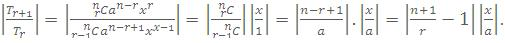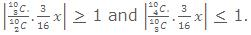×#### Thank you for registering.

One of our academic counsellors will contact you within 1 working day.

Click to Chat

1800-1023-196

+91-120-4616500

CART 0

• 0

MY CART (5)

Use Coupon: CART20 and get 20% off on all online Study Material

ITEM
DETAILS
MRP
DISCOUNT
FINAL PRICE
Total Price: Rs.

There are no items in this cart.
Continue Shopping```Greatest Binomial CoefficientTo determine the greatest coefficient in the binomial expansion, (1+x)n, when n is a positive integer. Coefficient of

(Tr+1/Tr) = Cr/Cr-1 = (n-r+1)/r = ((n+1)/r) - 1.

Now the (r+1)th binomial coefficient will be greater than the rth binomial coefficient when, Tr+1 > Tr

=>     ((n+1)/r)-1 > 1 => (n+1)/2 >r.                                   ....... (1)

But r must be an integer, and therefore when n is even, the greatest binomial coefficient is given by the greatest value of r, consistent with (1) i.e., r = n/2 and hence the greatest binomial coefficient is nCn/2.

Similarly in n be odd, the greatest binomial coefficient is given when,

r = (n-1)/2 or (n+1)/2 and the coefficient itself will be nC(n+1)/2 or nC(n-1)/2, both being are equal

Note: The greatest binomial coefficient is the binomial coefficient of the middle term.

Illustration:

Show that the greatest the coefficient in the expansion of (x + 1/x)2n is (1.3.5...(2n-1).2n)/n!  .

Solution:

Since middle term has the greatest coefficient.

So, greatest coefficient = coefficient of middle term

= 2nCn = (1.2.3...2n)/n!n! = (1.3.5...(2n-1).2n)/n!.

Numerically greatest term

To determine the numerically greatest term in the expansion of (a + x)n, where n is a positive integer.

ConsiderThusNote : {((n+1)/r) - 1} must be positive since n > r. Thus Tr+1 will be the greatest term if, r has the greatest value as per the equation (1).

Illustration:

Find the greatest term in the expansion of (3-2x)9 when x = 1.

Solution:

Tr+1/Tr = ((9-r+1)/r) . (2x/3) >1

i.e. 20 > 5r

If r = 4, then Tr+1 = Tr and these are the greatest terms. Thus 4th and 5th terms are numerically equal and greater than any other term and their value is equal 39 × 9C3 × (2/3)3 = 489888.

Illustration:

Find the greatest term in the expansion of (2 + 3x)9 if x = 3/2.

Solution:

Here  Tr+1 /Tr = ((n-r+1)/r)(3x/2) = ((10-r)/r)(3x/2), (where x = 3/2)

= ((10-r)/r)(3x/2)(3/2) = ((10-r)/r).9/4 = (90-9r)/4r

Therefore Tr+1 > Tr, if 90 - 9r > 4r.

=> 90 > 13r => r < 90/13 and r being an integer, r = 6.

Hence Tr+1 = T7 = T6+1 = 9C6 (2)3 (3x)6 = 313.7/2.

Illustration:

Given that the 4th term in the expansion of (2 + (3/8)x)10 has the maximum numerical value, find the range of values of x for which this will be true.

Solution:

Given 4th term in (2 + (3/8)x)10 = 210 (1 + (3/16)x)10, is numerically greatest

=>|T4/T3| > 1 and |T5/T4| < 1

=>=>|x| > 2 and |x| < 64/21

=> x ε [-(64/21),-2]U[2,(64/21)].

Illustration:

Find the greatest term in the expansion of √3(1 + (1/√3))20.

Solution:

Let rth term be the greatest

Since Tr/Tr+1 = (r/(21-r)).√3.

Now Tr/Tr+1 > 1 => r > 21/(√3+1)                                             ...... (1)

Now again Tr/Tr+1 = (r/(21-r)) √3 < 1 => r <  (22+√3)/(√3+1)               ...... (2)

from (1) and (2)

22/(√3+1) < r < (22+√3)/(√3+1)

=> r = 8 is the greatest term and its value is √3 . 20C7­ (1/√3)7 = 20C7  (1/27).

Particular Cases

We have (a + x)n = an + nC1 an-1 x + nC2 an-2 xr +...+ xn.            ...... (1)

(i) Putting x = -x in (1), we get

(a-x)n = an - nC1 an-1x + nC2 an-2 x2 - nC2 an-3 x3 +...+ (-1)r nCr an-r xr +...+ (-1)n xn.

(ii) Putting a = 1 in (1), we get,

(1+x)n = nC0 + nC1x + nC2x2 +...+ nCr xr +...+ nCnxn.                    ... (A)

(iii) Putting a = 1, x = -x in (1), we get

(1-x)n=nC0-nC1 x + nC2 x2-nC3x3 +... (-1)r nCr xr +... (-1)n nCnxn     ... (B)

Tips to Remember

(a) Tr/Tr+1 = ((n-r+1)/r ).(x/a) for the binomial expansion of (a + x)n.

(b) (n+1)Cr = nCr + nCr-1.

(c) r nCr = n n-1Cr-1

(d) When n is even,

(x + a)n + (x - a)n = 2(xn + nC2 xn-2 a2 + nC4 xn-4 a4 +...+ nCn an).

When n is odd,

(x + a)n + (x - a)n = 2(xn + nC2 xn-2 a2 +...+ nCn-1 x an-1).

When n is even

(x + a)n - (x - a)n = 2(nC1 xn-1 a + nC3 xn-3 a3 +...+ nCn-1 x an-1).

When n is odd

(x + a)n - (x - a)n = 2(nC1 xn-1 a + nC3 xn-3 a3 +...+ nCn an).

Properties of Binomial Coefficients

For the sake of convenience, the coefficients nCo, nC1, ..., nCr, ..., nCn are usually denoted by Co, C1,..., Cr, ..., Cn respectively

Put x = 1 in (A) and get, 2n = Co + C1 + ... + Cn                       ... (D)

Also putting x = -1 in (A) we get,

0 = Co - C1 + C2 - C3 + ......

=> C0 + C2 + C4 + ...... + C1 + C3 + C5 +...... = 2n.

Hence Co + C2 + C4 +...... = C1 + C3 + C5 +...... = 2n-1.

Illustration:

If (1 + x)n = C0 + C1x + C2x2 +...+ Cnxn, then prove that C0 + (Co + C1) + (C0 + C1 + C2) + ... (C0 + C1 + C2 +...+ Cn-1) = n2n-1 (where n is an even integer.

Solution:

C0 + (C0 + C1) + (C0 + C1 + C2) +... (C0 + C1 + C2 +...+ Cn-1)

= C0+(C0 + C1 + C2 +...+ Cn-1)+(C0 + C1)+(C0 + C1 + C2 +...+ Cn-2)+...

= (C0 + C1 + C2 +...+ Cn)+ (C0 + C1 + C2 +...+ Cn)+... n/2 times

= (n/2)2n = n . 2n-1

Free study material for the preparation of IIT JEE, AIEEE and other engineering examinations is available online at askIITians.com. Study Physics, Chemistry and Mathematics at askIITians website and be a winner. We offer numerous live online courses as well for live online IIT JEE preparation - you do not need to travel anywhere any longer - just sit at your home and study for IIT JEE live online with askIITians.com

To read more, Buy study materials of Binomial Theorem comprising study notes, revision notes, video lectures, previous year solved questions etc. Also browse for more study materials on Mathematics here.
```### Course Features

• 731 Video Lectures
• Revision Notes
• Previous Year Papers
• Mind Map
• Study Planner
• NCERT Solutions
• Discussion Forum
• Test paper with Video Solution